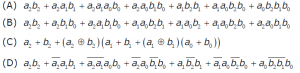GeeksforGeeks App
Open AppBrowser
Continue

# GATE | GATE-CS-2006 | Question 85

Given two three bit numbers a2a1a0 and b2b1b0 and c, the carry in, the function that represents the carry generate function when these two numbers are added is:(A) A
(B) B
(C) C
(D) D

Where

As we are having two 3 bits number to add so final carry out will be C3-

Putting value of Pi,Gi in 3

C3=(A2.B2)+(A1.B1)(A2+B2)+(A0.B0)(A1+B1)(A2+B2)                       (TAKING C0=0)

C3=A2.B2 +A1A2B1+A1B2B1+(A0B0)(A1A2+A1B2+B1A2+B1B2)

C3=A2B2+A1A2B1+A1B2B1+A0A1A2B0+A0A1B0B2+A0A2B1B0+A0B0B1B2

SO ANS IS (A) PART.

Quiz of this Question

My Personal Notes arrow_drop_up